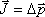This is a big one! It is the Impulse-Momentum Relationship. It states that the net impulse delivered to an object is equal to the change in momentum of that object.

Tip: This is a cause equals effect equation. The cause on the left is equal to the effect on the right. When you use this equation, after first writing it down, you should enter into substitution mode. On the left, substitute the applicable net force-times-time-interval for the J; on the right, substitute the final momentum minus the initial momentum. Keep the cause on the left and the effect on the right until you have boiled it down to an algebra problem which you can then solve for the sought quantity.

Tip: The impulse in this equation is the net impulse. In the case of more than one force acting on an object, the net impulse can be determined by finding the net force and multiplying that by the time interval during which it acts (see the definition of impulse), or, the net impulse can be determined by finding the impulse due to each force (again using the definition of impulse) and adding all the impulses together like vectors.

Pitfall Avoidance Note: Both impulse and momentum have direction. In problems involving motion in one dimension, there are only two possible directions so you can call one direction the positive direction and the other the negative direction. Establish a positive direction and stick with it throughout the problem on both sides of the equation.